# RS Aggarwal Solutions for Class 7 Maths Exercise 10A Chapter 10 Percentage

RS Aggarwal Solutions for Exercise 10A of Class 7 Maths Chapter 10 Percentage. This exercise contains conversion of fraction into a percentage, conversion of percentage into a fraction, percentage as ratio, conversion of ratio into a percentage, conversion of percentage into decimal form, conversion of decimal into a percentage. Solutions are prepared by expert personnel with a neat description. Students can go through this RS Aggarwal Solutions for Class 7 Maths Chapter 10 Percentage and practice more problems. Students who wish to score good marks in Maths practise RS Aggarwal Class 7 Solutions Chapter 10. Our expert professors formulate these exercises to support you with your exam preparation to score good marks in Maths

## Download the PDF of RS Aggarwal Solutions For Class 7 Maths Chapter 10 Percentage – Exercise 10A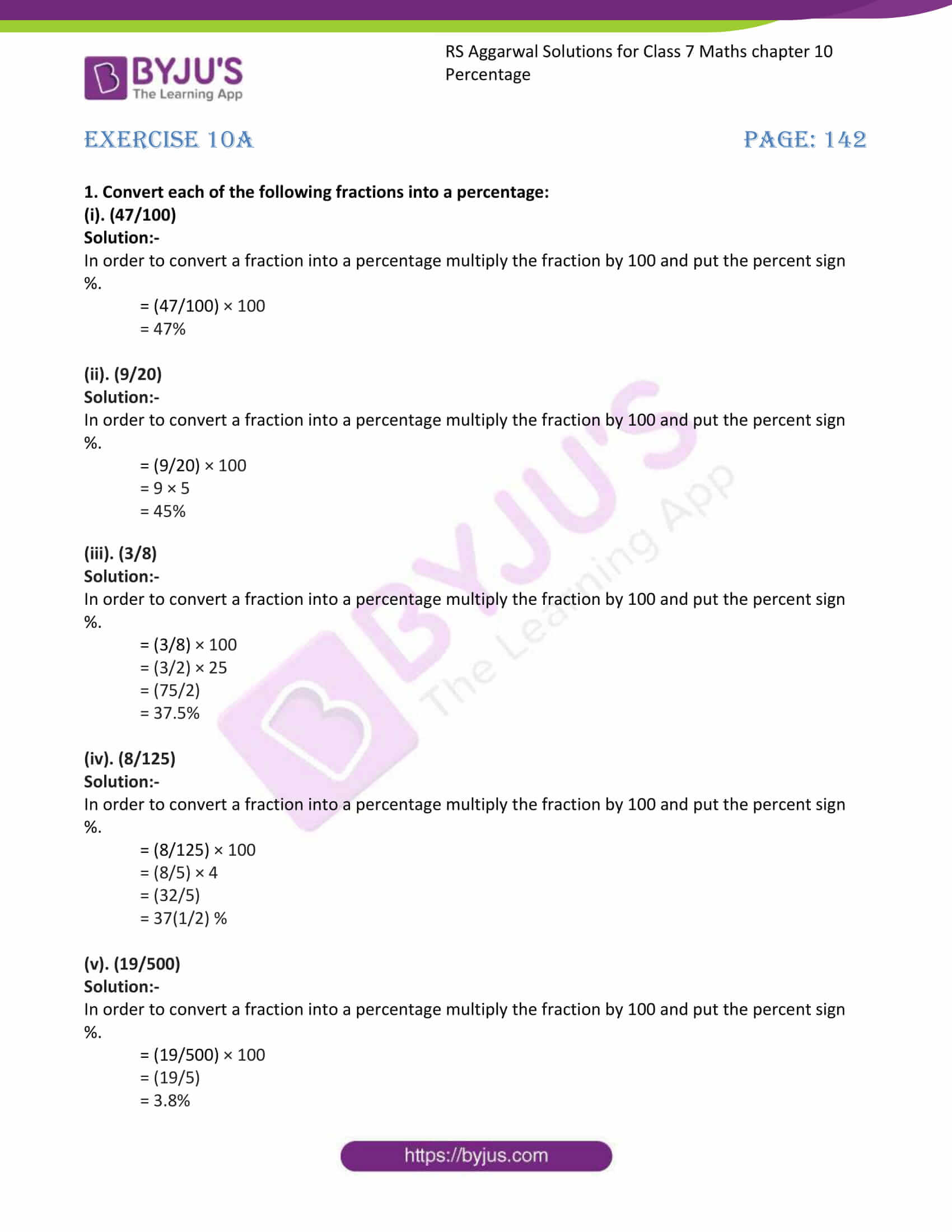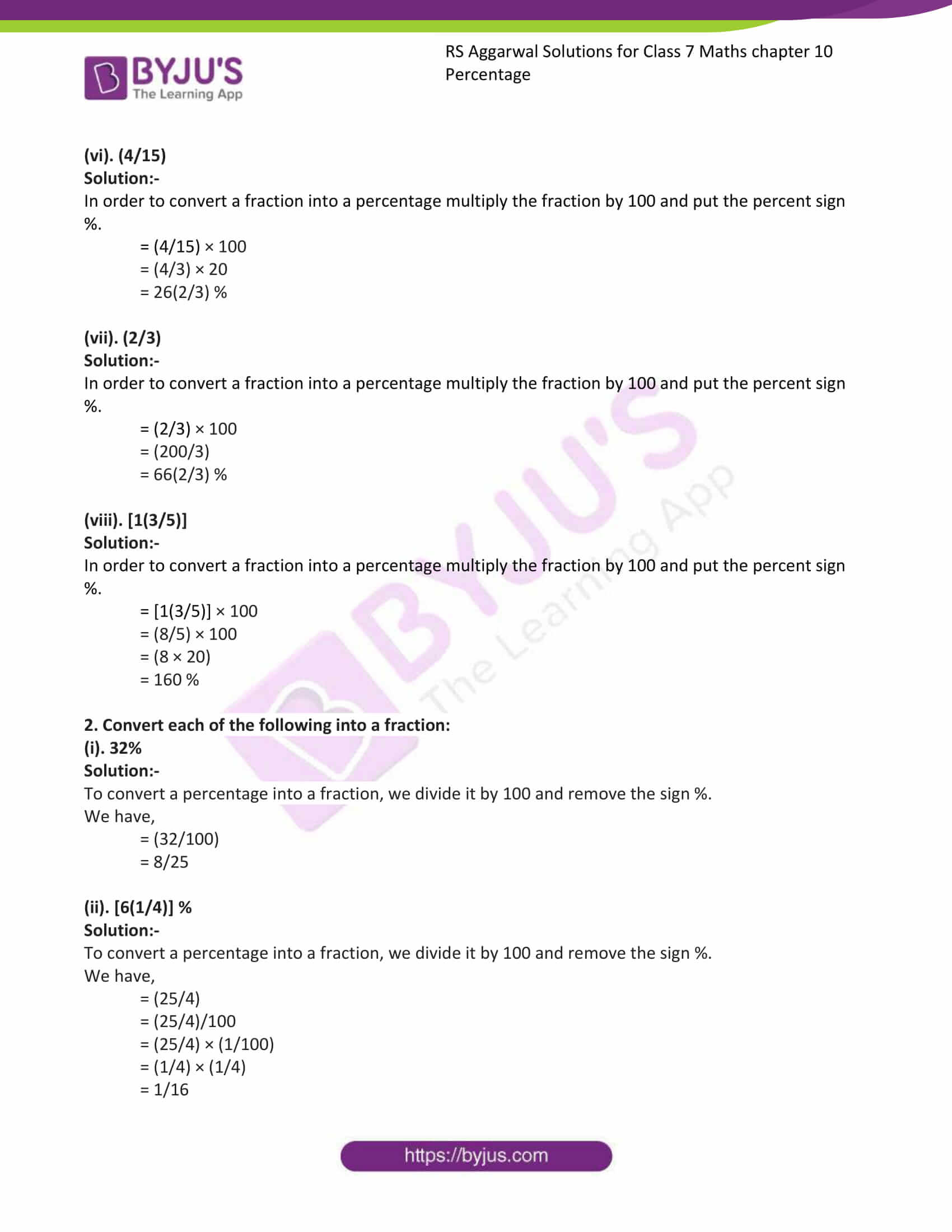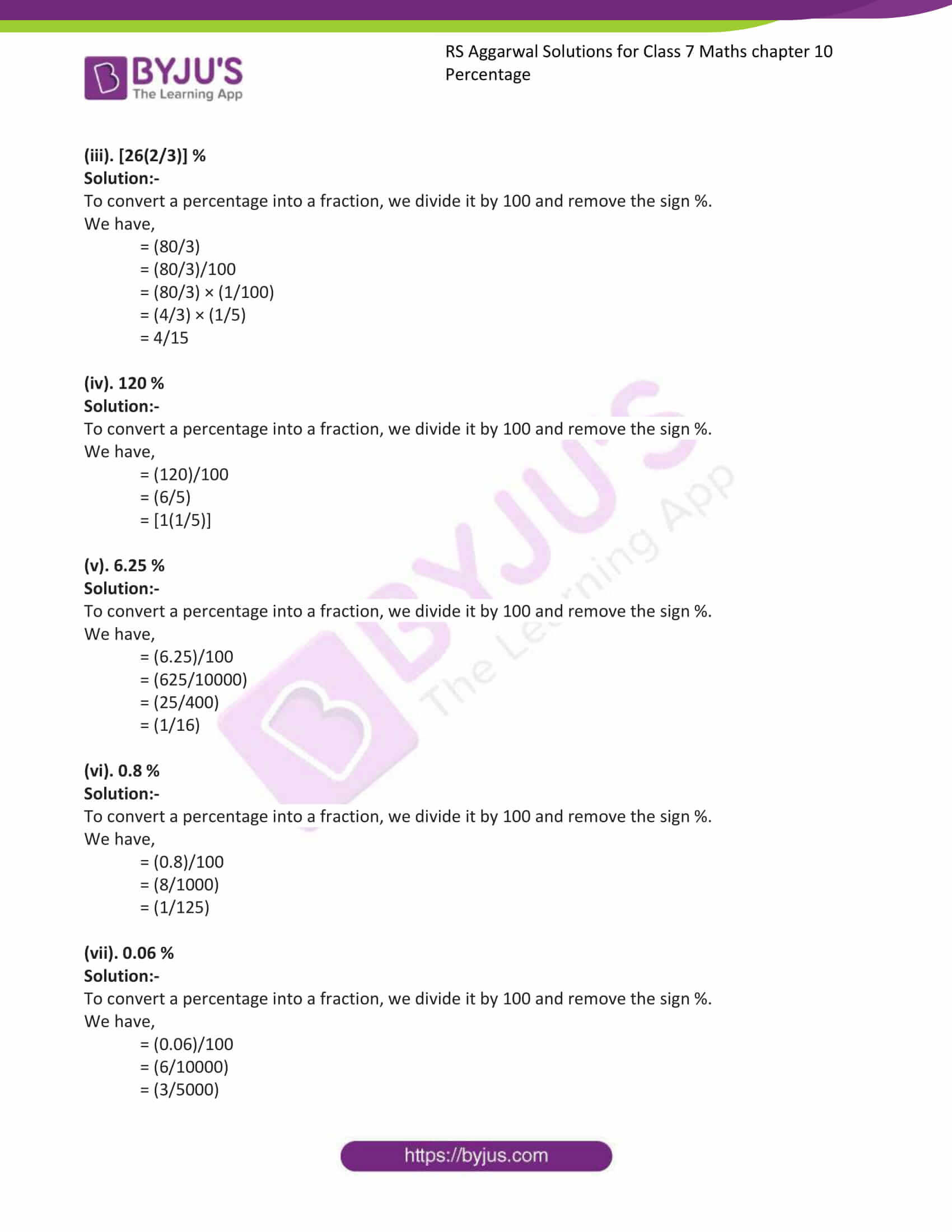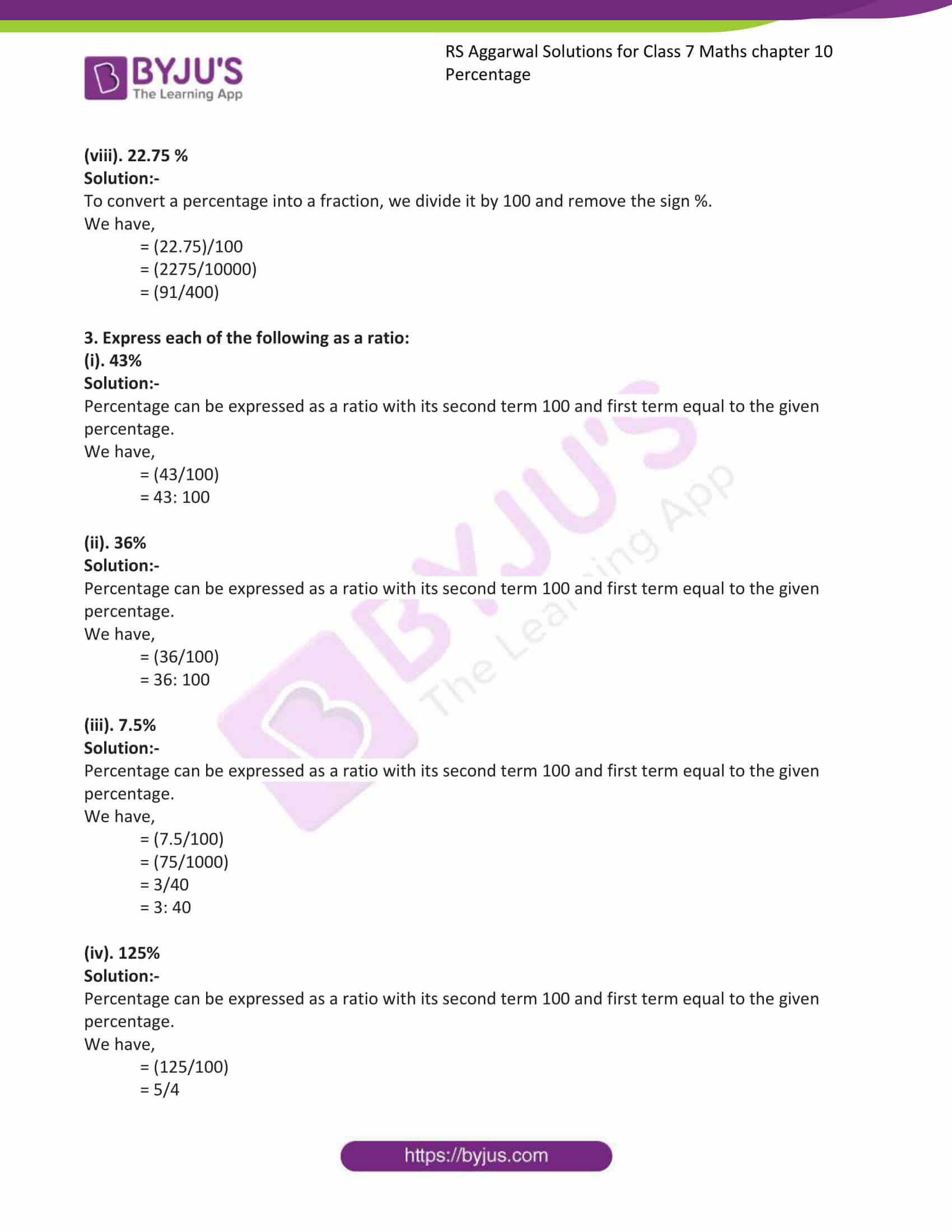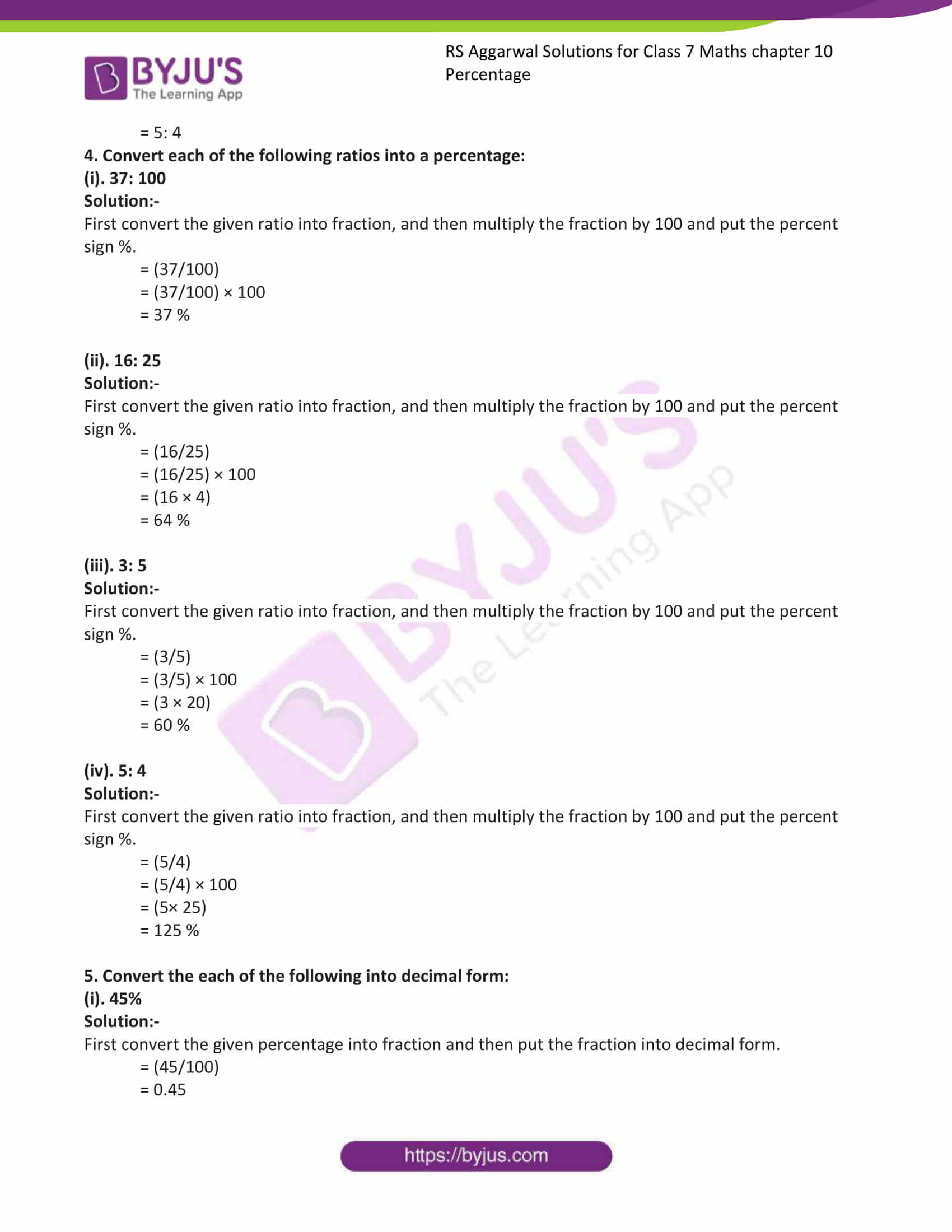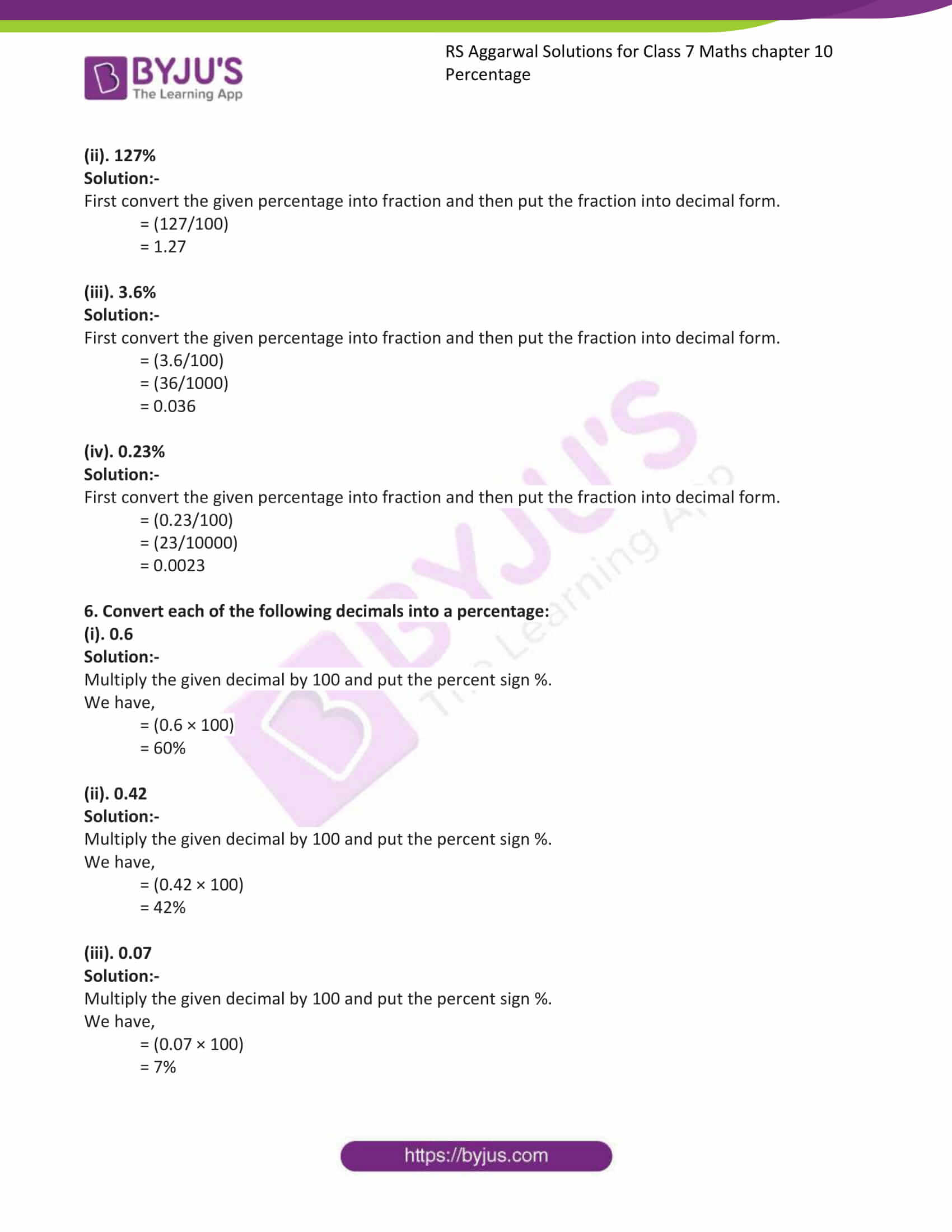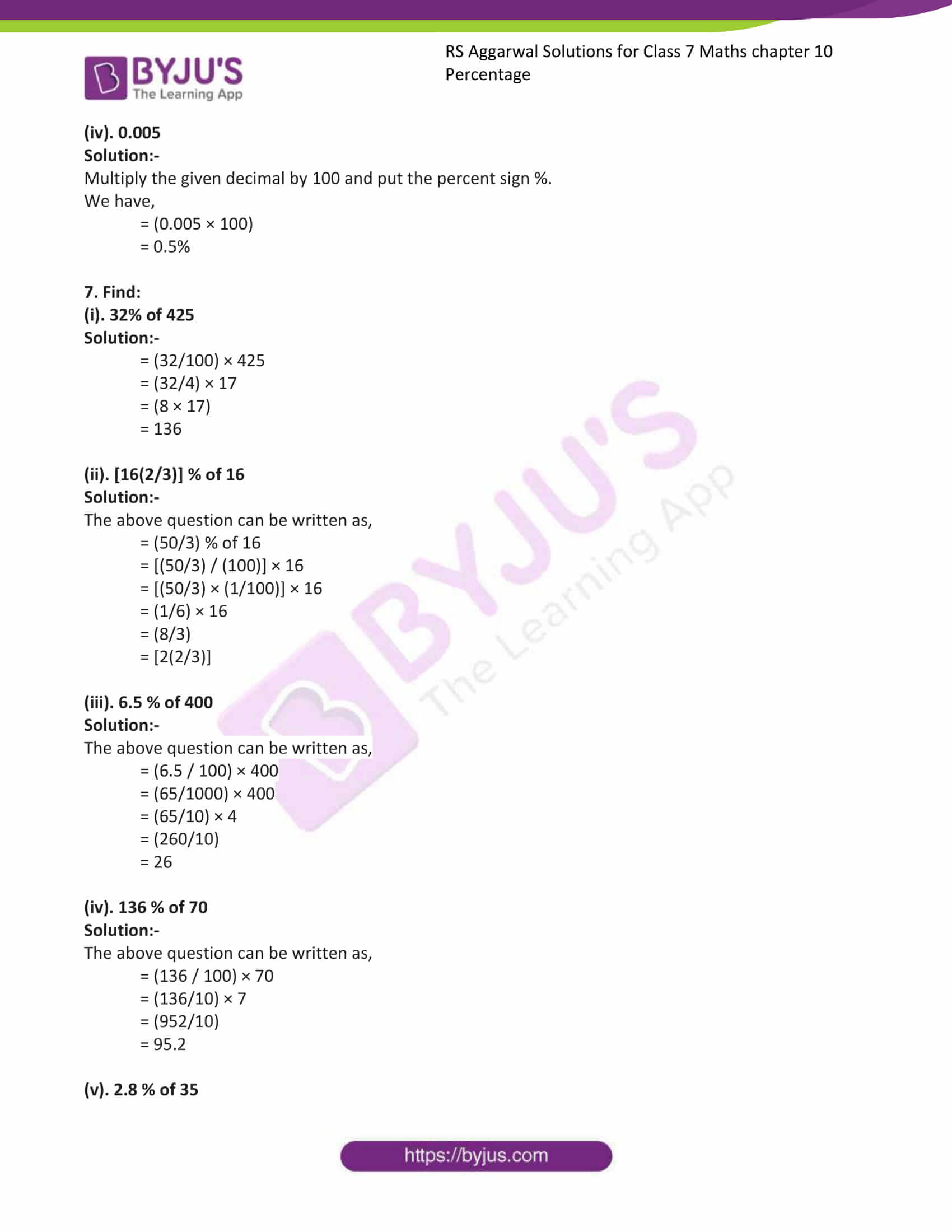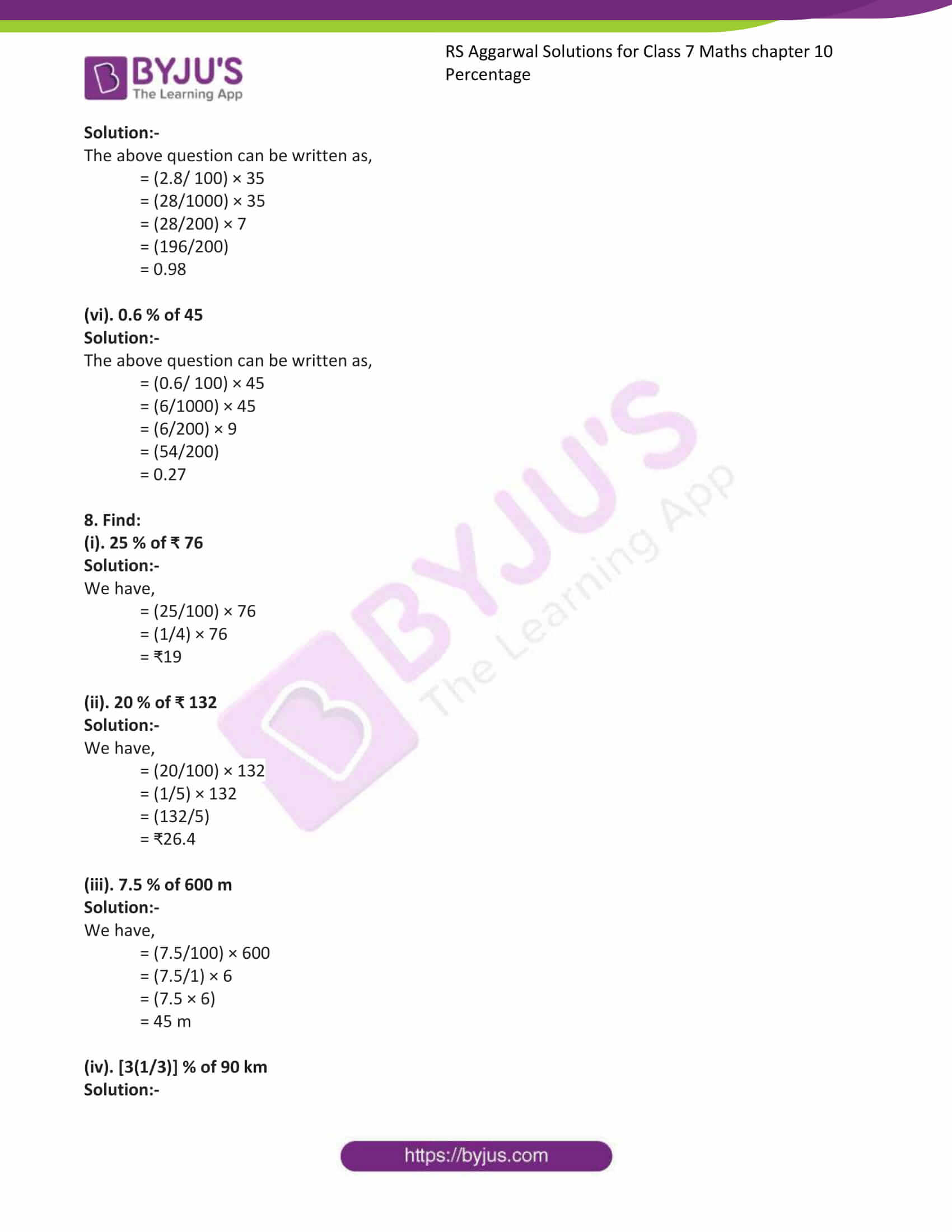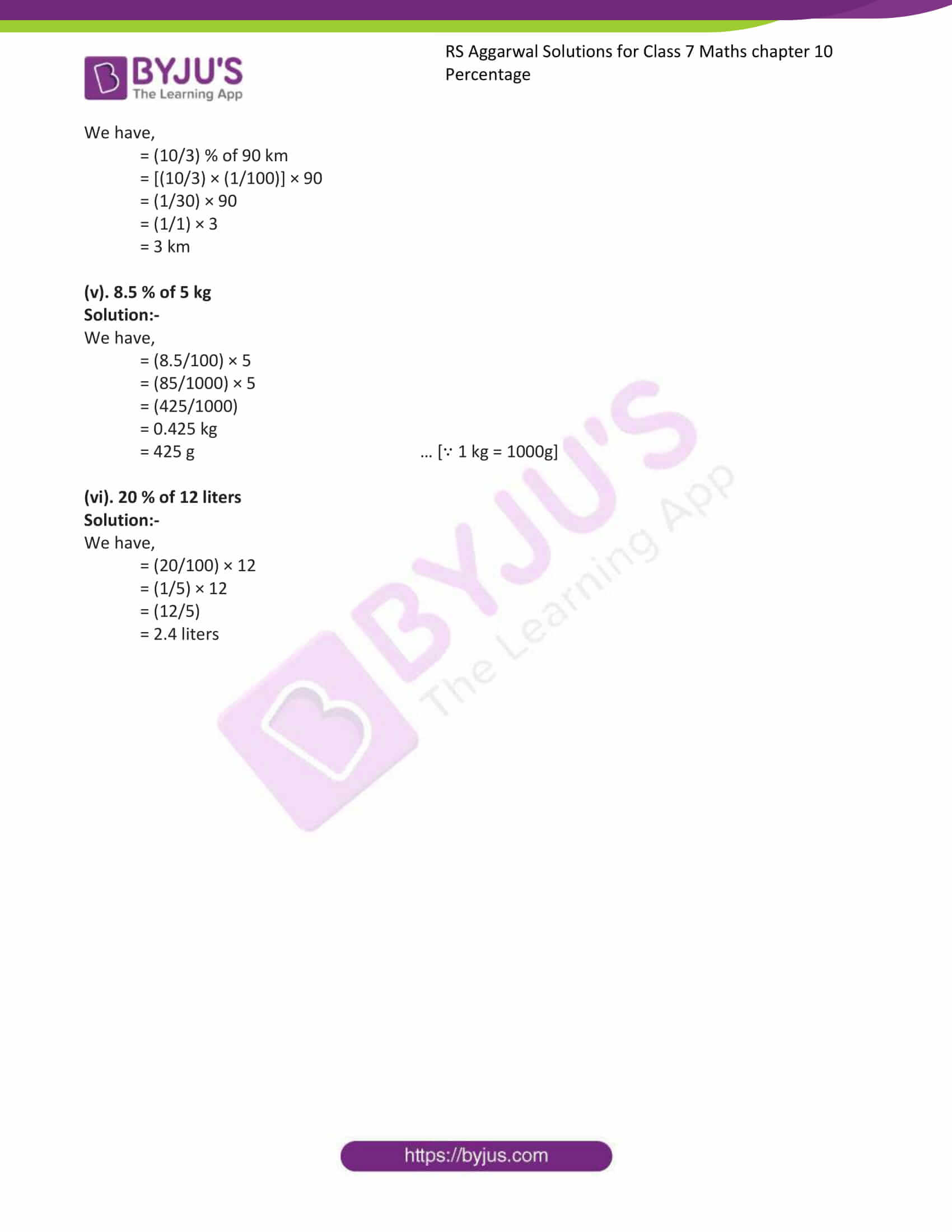### Access answers to Maths RS Aggarwal Solutions for Class 7 Chapter 10 – Percentage Exercise 10A

1. Convert each of the following fractions into a percentage:

(i). (47/100)

Solution:-

In order to convert a fraction into a percentage multiply the fraction by 100 and put the percent sign %.

= (47/100) × 100

= 47%

(ii). (9/20)

Solution:-

In order to convert a fraction into a percentage multiply the fraction by 100 and put the percent sign %.

= (9/20) × 100

= 9 × 5

= 45%

(iii). (3/8)

Solution:-

In order to convert a fraction into a percentage multiply the fraction by 100 and put the percent sign %.

= (3/8) × 100

= (3/2) × 25

= (75/2)

= 37.5%

(iv). (8/125)

Solution:-

In order to convert a fraction into a percentage multiply the fraction by 100 and put the percent sign %.

= (8/125) × 100

= (8/5) × 4

= (32/5)

= 37(1/2) %

(v). (19/500)

Solution:-

In order to convert a fraction into a percentage multiply the fraction by 100 and put the percent sign %.

= (19/500) × 100

= (19/5)

= 3.8%

(vi). (4/15)

Solution:-

In order to convert a fraction into a percentage multiply the fraction by 100 and put the percent sign %.

= (4/15) × 100

= (4/3) × 20

= 26(2/3) %

(vii). (2/3)

Solution:-

In order to convert a fraction into a percentage multiply the fraction by 100 and put the percent sign %.

= (2/3) × 100

= (200/3)

= 66(2/3) %

(viii). [1(3/5)]

Solution:-

In order to convert a fraction into a percentage multiply the fraction by 100 and put the percent sign %.

= [1(3/5)] × 100

= (8/5) × 100

= (8 × 20)

= 160 %

2. Convert each of the following into a fraction:

(i). 32%

Solution:-

To convert a percentage into a fraction, we divide it by 100 and remove the sign %.

We have,

= (32/100)

= 8/25

(ii). [6(1/4)] %

Solution:-

To convert a percentage into a fraction, we divide it by 100 and remove the sign %.

We have,

= (25/4)

= (25/4)/100

= (25/4) × (1/100)

= (1/4) × (1/4)

= 1/16

(iii). [26(2/3)] %

Solution:-

To convert a percentage into a fraction, we divide it by 100 and remove the sign %.

We have,

= (80/3)

= (80/3)/100

= (80/3) × (1/100)

= (4/3) × (1/5)

= 4/15

(iv). 120 %

Solution:-

To convert a percentage into a fraction, we divide it by 100 and remove the sign %.

We have,

= (120)/100

= (6/5)

= [1(1/5)]

(v). 6.25 %

Solution:-

To convert a percentage into a fraction, we divide it by 100 and remove the sign %.

We have,

= (6.25)/100

= (625/10000)

= (25/400)

= (1/16)

(vi). 0.8 %

Solution:-

To convert a percentage into a fraction, we divide it by 100 and remove the sign %.

We have,

= (0.8)/100

= (8/1000)

= (1/125)

(vii). 0.06 %

Solution:-

To convert a percentage into a fraction, we divide it by 100 and remove the sign %.

We have,

= (0.06)/100

= (6/10000)

= (3/5000)

(viii). 22.75 %

Solution:-

To convert a percentage into a fraction, we divide it by 100 and remove the sign %.

We have,

= (22.75)/100

= (2275/10000)

= (91/400)

3. Express each of the following as a ratio:

(i). 43%

Solution:-

Percentage can be expressed as a ratio with its second term 100 and first term equal to the given percentage.

We have,

= (43/100)

= 43: 100

(ii). 36%

Solution:-

Percentage can be expressed as a ratio with its second term 100 and first term equal to the given percentage.

We have,

= (36/100)

= 36: 100

(iii). 7.5%

Solution:-

Percentage can be expressed as a ratio with its second term 100 and first term equal to the given percentage.

We have,

= (7.5/100)

= (75/1000)

= 3/40

= 3: 40

(iv). 125%

Solution:-

Percentage can be expressed as a ratio with its second term 100 and first term equal to the given percentage.

We have,

= (125/100)

= 5/4

= 5: 4

4. Convert each of the following ratios into a percentage:

(i). 37: 100

Solution:-

First convert the given ratio into fraction, and then multiply the fraction by 100 and put the percent sign %.

= (37/100)

= (37/100) × 100

= 37 %

(ii). 16: 25

Solution:-

First convert the given ratio into fraction, and then multiply the fraction by 100 and put the percent sign %.

= (16/25)

= (16/25) × 100

= (16 × 4)

= 64 %

(iii). 3: 5

Solution:-

First convert the given ratio into fraction, and then multiply the fraction by 100 and put the percent sign %.

= (3/5)

= (3/5) × 100

= (3 × 20)

= 60 %

(iv). 5: 4

Solution:-

First convert the given ratio into fraction, and then multiply the fraction by 100 and put the percent sign %.

= (5/4)

= (5/4) × 100

= (5× 25)

= 125 %

5. Convert the each of the following into decimal form:

(i). 45%

Solution:-

First convert the given percentage into fraction and then put the fraction into decimal form.

= (45/100)

= 0.45

(ii). 127%

Solution:-

First convert the given percentage into fraction and then put the fraction into decimal form.

= (127/100)

= 1.27

(iii). 3.6%

Solution:-

First convert the given percentage into fraction and then put the fraction into decimal form.

= (3.6/100)

= (36/1000)

= 0.036

(iv). 0.23%

Solution:-

First convert the given percentage into fraction and then put the fraction into decimal form.

= (0.23/100)

= (23/10000)

= 0.0023

6. Convert each of the following decimals into a percentage:

(i). 0.6

Solution:-

Multiply the given decimal by 100 and put the percent sign %.

We have,

= (0.6 × 100)

= 60%

(ii). 0.42

Solution:-

Multiply the given decimal by 100 and put the percent sign %.

We have,

= (0.42 × 100)

= 42%

(iii). 0.07

Solution:-

Multiply the given decimal by 100 and put the percent sign %.

We have,

= (0.07 × 100)

= 7%

(iv). 0.005

Solution:-

Multiply the given decimal by 100 and put the percent sign %.

We have,

= (0.005 × 100)

= 0.5%

7. Find:

(i). 32% of 425

Solution:-

= (32/100) × 425

= (32/4) × 17

= (8 × 17)

= 136

(ii). [16(2/3)] % of 16

Solution:-

The above question can be written as,

= (50/3) % of 16

= [(50/3) / (100)] × 16

= [(50/3) × (1/100)] × 16

= (1/6) × 16

= (8/3)

= [2(2/3)]

(iii). 6.5 % of 400

Solution:-

The above question can be written as,

= (6.5 / 100) × 400

= (65/1000) × 400

= (65/10) × 4

= (260/10)

= 26

(iv). 136 % of 70

Solution:-

The above question can be written as,

= (136 / 100) × 70

= (136/10) × 7

= (952/10)

= 95.2

(v). 2.8 % of 35

Solution:-

The above question can be written as,

= (2.8/ 100) × 35

= (28/1000) × 35

= (28/200) × 7

= (196/200)

= 0.98

(vi). 0.6 % of 45

Solution:-

The above question can be written as,

= (0.6/ 100) × 45

= (6/1000) × 45

= (6/200) × 9

= (54/200)

= 0.27

8. Find:

(i). 25 % of ₹ 76

Solution:-

We have,

= (25/100) × 76

= (1/4) × 76

= ₹19

(ii). 20 % of ₹ 132

Solution:-

We have,

= (20/100) × 132

= (1/5) × 132

= (132/5)

= ₹26.4

(iii). 7.5 % of 600 m

Solution:-

We have,

= (7.5/100) × 600

= (7.5/1) × 6

= (7.5 × 6)

= 45 m

(iv). [3(1/3)] % of 90 km

Solution:-

We have,

= (10/3) % of 90 km

= [(10/3) × (1/100)] × 90

= (1/30) × 90

= (1/1) × 3

= 3 km

(v). 8.5 % of 5 kg

Solution:-

We have,

= (8.5/100) × 5

= (85/1000) × 5

= (425/1000)

= 0.425 kg

= 425 g … [∵ 1 kg = 1000g]

(vi). 20 % of 12 liters

Solution:-

We have,

= (20/100) × 12

= (1/5) × 12

= (12/5)

= 2.4 liters

### Access other exercises of RS Aggarwal Solutions For Class 7 Chapter 10 – Percentage

Exercise 10B Solutions

Exercise 10C Solutions